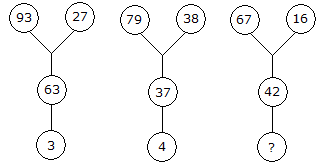# Verbal Reasoning - Character Puzzles - Discussion

### Discussion :: Character Puzzles - Character Puzzles 1 (Q.No.31)

31.

Which one will replace the question mark ?[A]. 5 [B]. 6 [C]. 8 [D]. 9

Explanation:

93 - (27 + 3) = 63

79 - (38 + 4) = 37

Therefore, 67 - (16 + X) = 42

X = 9

 Puja said: (May 20, 2011) 27+63+3=93 38+37+4=79 16+42+?=67 ans is 9

 Ssv said: (Nov 9, 2013) Look at the arrows they tell the whole story: 93-27-3= 63. 79-38-4= 37. 67-16 - ? = 42. X = 67-16-42. = 9.

 Mounika said: (Sep 27, 2016) Substract top (93 - 27) = 66 - 63 = 3, (79 - 38) = 41 - 37 = 4, (67 - 16) = 51 - 42 = 9.

 Shagun said: (Jun 27, 2017) 93-63-27=3. 79-37-38=4. 67-42-16=9.

 Ashmit said: (Jul 21, 2018) 93-27-3 = 63. 79-38-4 = 37. 67-16-9 = 42.

 Deblina said: (Jul 17, 2019) 93-27 = 66-63 = 3 79-38 = 41-37 = 4 67-16 = 51-42 = 9.

 H.D. said: (Dec 15, 2020) 93 - 27 = 66 - 3 = 63. 79 - 38 = 41 - 4 = 37. And so, 67 -16 = 51 - 42 = 9.

 Kavitha said: (Jan 16, 2021) 93-27-3 = 30. 79-38-4 = 37. And also; 67 - 16 - X = 42, 51-X=42, -X =-51 + 42, -X = -9, X = 9.

 Swati said: (Feb 13, 2021) 93 - (63 + 27) = 3, 79 - (38 + 37) = 4, 67 - (16 + 42) = 9, So, 9 is the answer.

 Babor said: (Apr 5, 2021) (27+63)-93 = 3. (38+37)-79 = 4. (42+16)-67 = 9.

 Naveen Kuamr said: (May 11, 2021) Thanks all for explaining.